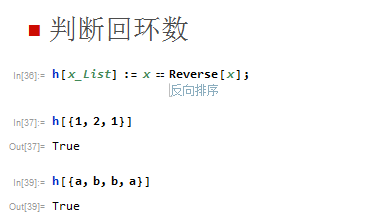Mathematica函数的参数设定|回环数例子

• A+

Mathematica实现回环数

``h[x_List] := x == Reverse[x];``• 微信公众号
• 关注微信公众号
•• QQ群
• 我们的QQ群号
•